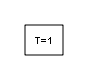Timed-Based Linearization

Generate linear models in base workspace at specific times

• Library:

•Description

This block calls linmod or dlinmod to create a linear model for the system when the simulation clock reaches the time specified by the Linearization time parameter. No trimming is performed. The linear model is stored in the base workspace as a structure, along with information about the operating point at which the snapshot was taken. Multiple snapshots are appended to form an array of structures.

The block sets the following model parameters to the indicated values:

• BufferReuse = 'off'

• RTWInlineParameters = 'on'

• BlockReductionOpt = 'off'

The name of the structure used to save the snapshots is the name of the model appended by _Timed_Based_Linearization, for example, vdp_Timed_Based_Linearization. The structure has the following fields:

FieldDescription

a

The A matrix of the linearization

b

The B matrix of the linearization

c

The C matrix of the linearization

d

The D matrix of the linearization

StateName

Names of the model's states

OutputName

Names of the model's output ports

InputName

Names of the model's input ports

OperPoint

A structure that specifies the operating point of the linearization. The structure specifies the operating point time (OperPoint.t). The states (OperPoint.x) and inputs (OperPoint.u) fields are not used.

Ts

The sample time of the linearization for a discrete linearization

Tip

To generate models conditionally, use the Trigger-Based Linearization block.

Parameters

expand all

Time at which you want the block to generate a linear model. Enter a vector of times if you want the block to generate linear models at more than one time step.

Programmatic Use

 Block Parameter: LinearizationTime Type: character vector Values: scalar | vector Default: '1'

Specify a sample time for the linear model. To create a continuous-time model using linmod, specify a sample time of 0. Otherwise, to create a discrete-time model using dlinmod, specify a positive sample time (see Discrete-Time System Linearization).

Programmatic Use

 Block Parameter: SampleTime Type: character vector Values: scalar | vector Default: '0'

Block Characteristics

 Data Types Direct Feedthrough no Multidimensional Signals no Variable-Size Signals no Zero-Crossing Detection no